# Selecting rows in pandas DataFrame based on conditions

Let’s see how to Select rows based on some conditions in Pandas DataFrame.

### Selecting rows based on particular column value using `'>', '=', '=', '<=', '!=' `operator.

Code #1 : Selecting all the rows from the given dataframe in which ‘Percentage’ is greater than 80 using basic method.

Python3

``````
# importing pandas
import pandas as pd

record = {

'Name': ['Ankit', 'Amit', 'Aishwarya', 'Priyanka', 'Priya', 'Shaurya' ],
'Age': [21, 19, 20, 18, 17, 21],
'Stream': ['Math', 'Commerce', 'Science', 'Math', 'Math', 'Science'],
'Percentage': [88, 92, 95, 70, 65, 78] }

# create a dataframe
dataframe = pd.DataFrame(record, columns = ['Name', 'Age', 'Stream', 'Percentage'])

print(&quot;Given Dataframe :\n&quot;, dataframe)

# selecting rows based on condition
rslt_df = dataframe[dataframe['Percentage'] &gt; 80]

print('\nResult dataframe :\n', rslt_df)
``````

Output :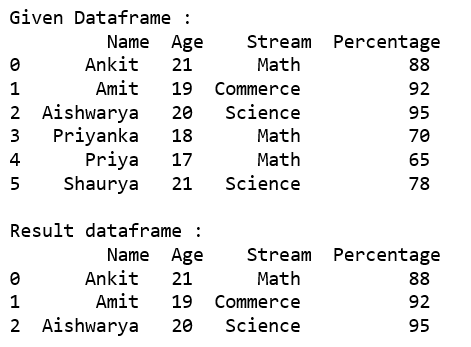Code #2 : Selecting all the rows from the given dataframe in which ‘Percentage’ is greater than 80 using loc.

Python3

``````
# importing pandas
import pandas as pd

record = {
'Name': ['Ankit', 'Amit', 'Aishwarya', 'Priyanka', 'Priya', 'Shaurya' ],
'Age': [21, 19, 20, 18, 17, 21],
'Stream': ['Math', 'Commerce', 'Science', 'Math', 'Math', 'Science'],
'Percentage': [88, 92, 95, 70, 65, 78]}

# create a dataframe
dataframe = pd.DataFrame(record, columns = ['Name', 'Age', 'Stream', 'Percentage'])

print(&quot;Given Dataframe :\n&quot;, dataframe)

# selecting rows based on condition
rslt_df = dataframe.loc[dataframe['Percentage'] &gt; 80]

print('\nResult dataframe :\n', rslt_df)
``````

Output :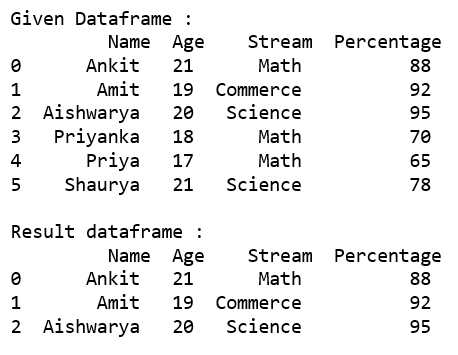Code #3 : Selecting all the rows from the given dataframe in which ‘Percentage’ is not equal to 95 using Loc.

Python3

``````
# importing pandas
import pandas as pd

record = {
'Name': ['Ankit', 'Amit', 'Aishwarya', 'Priyanka', 'Priya', 'Shaurya' ],
'Age': [21, 19, 20, 18, 17, 21],
'Stream': ['Math', 'Commerce', 'Science', 'Math', 'Math', 'Science'],
'Percentage': [88, 92, 95, 70, 65, 78]}

# create a dataframe
dataframe = pd.DataFrame(record, columns = ['Name', 'Age', 'Stream', 'Percentage'])

print(&quot;Given Dataframe :\n&quot;, dataframe)

# selecting rows based on condition
rslt_df = dataframe.loc[dataframe['Percentage'] != 95]

print('\nResult dataframe :\n', rslt_df)
``````

Output :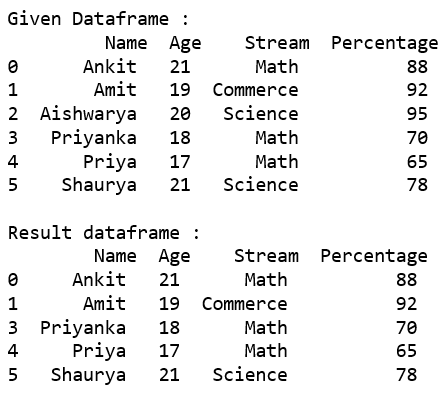### Selecting those rows whose column value is present in the list using `isin()` method of the dataframe.

Code #1 : Selecting all the rows from the given dataframe in which ‘Stream’ is present in the options list using basic method.

Python3

``````
# importing pandas
import pandas as pd

record = {
'Name': ['Ankit', 'Amit', 'Aishwarya', 'Priyanka', 'Priya', 'Shaurya' ],
'Age': [21, 19, 20, 18, 17, 21],
'Stream': ['Math', 'Commerce', 'Science', 'Math', 'Math', 'Science'],
'Percentage': [88, 92, 95, 70, 65, 78]}

# create a dataframe
dataframe = pd.DataFrame(record, columns = ['Name', 'Age', 'Stream', 'Percentage'])

print(&quot;Given Dataframe :\n&quot;, dataframe)

options = ['Math', 'Commerce']

# selecting rows based on condition
rslt_df = dataframe[dataframe['Stream'].isin(options)]

print('\nResult dataframe :\n', rslt_df)
``````

Output :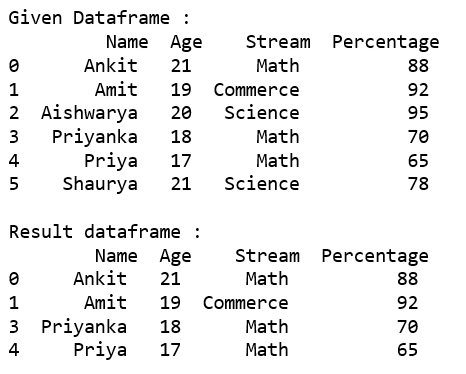Code #2 :

Selecting all the rows from the given dataframe in which ‘Stream’ is present in the options list using .loc[].

Python3

``````
# importing pandas
import pandas as pd

record = {
'Name': ['Ankit', 'Amit', 'Aishwarya', 'Priyanka', 'Priya', 'Shaurya' ],
'Age': [21, 19, 20, 18, 17, 21],
'Stream': ['Math', 'Commerce', 'Science', 'Math', 'Math', 'Science'],
'Percentage': [88, 92, 95, 70, 65, 78]}

# create a dataframe
dataframe = pd.DataFrame(record, columns = ['Name', 'Age', 'Stream', 'Percentage'])

print(&quot;Given Dataframe :\n&quot;, dataframe)

options = ['Math', 'Commerce']

# selecting rows based on condition
rslt_df = dataframe.loc[dataframe['Stream'].isin(options)]

print('\nResult dataframe :\n', rslt_df)
``````

Output :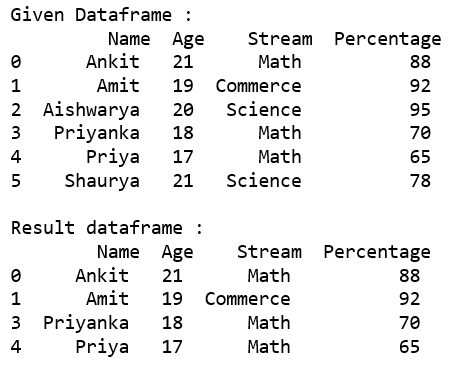Code #3 : Selecting all the rows from the given dataframe in which ‘Stream’ is not present in the options list using `.loc[]`.

Python3

``````
# importing pandas
import pandas as pd

record = {
'Name': ['Ankit', 'Amit', 'Aishwarya', 'Priyanka', 'Priya', 'Shaurya' ],
'Age': [21, 19, 20, 18, 17, 21],
'Stream': ['Math', 'Commerce', 'Science', 'Math', 'Math', 'Science'],
'Percentage': [88, 92, 95, 70, 65, 78]}

# create a dataframe
dataframe = pd.DataFrame(record, columns = ['Name', 'Age', 'Stream', 'Percentage'])

print(&quot;Given Dataframe :\n&quot;, dataframe)

options = ['Math', 'Science']

# selecting rows based on condition
rslt_df = dataframe.loc[~dataframe['Stream'].isin(options)]

print('\nresult dataframe :\n', rslt_df)
``````

Output :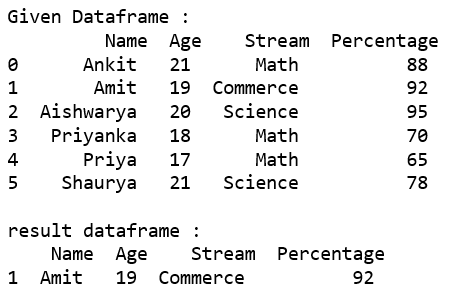### Selecting rows based on multiple column conditions using `'&'` operator.

Code #1 : Selecting all the rows from the given dataframe in which ‘Age’ is equal to 21 and ‘Stream’ is present in the options list using basic method.

Python3

``````
# importing pandas
import pandas as pd

record = {
'Name': ['Ankit', 'Amit', 'Aishwarya', 'Priyanka', 'Priya', 'Shaurya' ],
'Age': [21, 19, 20, 18, 17, 21],
'Stream': ['Math', 'Commerce', 'Science', 'Math', 'Math', 'Science'],
'Percentage': [88, 92, 95, 70, 65, 78]}

# create a dataframe
dataframe = pd.DataFrame(record, columns = ['Name', 'Age', 'Stream', 'Percentage'])

print(&quot;Given Dataframe :\n&quot;, dataframe)

options = ['Math', 'Science']

# selecting rows based on condition
rslt_df = dataframe[(dataframe['Age'] == 21) &amp;
dataframe['Stream'].isin(options)]

print('\nResult dataframe :\n', rslt_df)
``````

Output :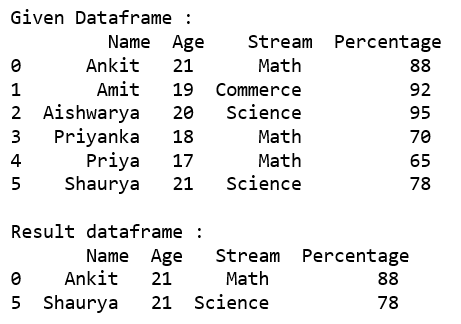Code #2 :

Selecting all the rows from the given dataframe in which ‘Age’ is equal to 21 and ‘Stream’ is present in the options list using

.loc[].

Python3

``````
# importing pandas
import pandas as pd

record = {
'Name': ['Ankit', 'Amit', 'Aishwarya', 'Priyanka', 'Priya', 'Shaurya' ],
'Age': [21, 19, 20, 18, 17, 21],
'Stream': ['Math', 'Commerce', 'Science', 'Math', 'Math', 'Science'],
'Percentage': [88, 92, 95, 70, 65, 78]}

# create a dataframe
dataframe = pd.DataFrame(record, columns = ['Name', 'Age', 'Stream', 'Percentage'])

print(&quot;Given Dataframe :\n&quot;, dataframe)

options = ['Math', 'Science']

# selecting rows based on condition
rslt_df = dataframe.loc[(dataframe['Age'] == 21) &amp;
dataframe['Stream'].isin(options)]

print('\nResult dataframe :\n', rslt_df)
``````

Output :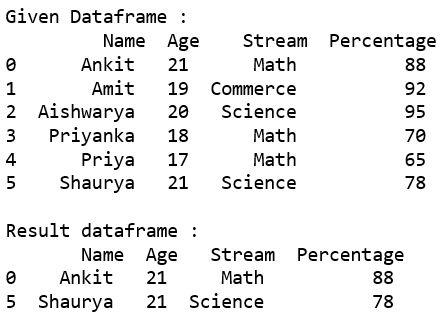Whether you're preparing for your first job interview or aiming to upskill in this ever-evolving tech landscape, GeeksforGeeks Courses are your key to success. We provide top-quality content at affordable prices, all geared towards accelerating your growth in a time-bound manner. Join the millions we've already empowered, and we're here to do the same for you. Don't miss out - check it out now!

Previous
Next